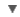HYPHEN
WORDNET DICTIONARY
CIDE DICTIONARY
Link, Gadget and Share#geometrical

RELATED WORDS :

:
ge=o=met=ri=cal

## CIDE DICTIONARY

geometricala. [L. geometricus; Gr. : cf. F. géométrique.].
•  Pertaining to, or according to the rules or principles of, geometry; determined by geometry; as, a geometrical solution of a problem.  [1913 Webster]
•  characterized by simple geometric forms in design and decoration; as, a buffalo hide painted with red and black geometrical designs.  [WordNet 1.5]
" Geometric is often used, as opposed to algebraic, to include processes or solutions in which the propositions or principles of geometry are made use of rather than those of algebra."  [1913 Webster]
" Geometrical is often used in a limited or strictly technical sense, as opposed to mechanical; thus, a construction or solution is geometrical which can be made by ruler and compasses, i. e., by means of right lines and circles. Every construction or solution which requires any other curve, or such motion of a line or circle as would generate any other curve, is not geometrical, but mechanical. By another distinction, a geometrical solution is one obtained by the rules of geometry, or processes of analysis, and hence is exact; while a mechanical solution is one obtained by trial, by actual measurements, with instruments, etc., and is only approximate and empirical."  [1913 Webster]
Geometrical curve. Same as Algebraic curve; -- so called because their different points may be constructed by the operations of elementary geometry. -- Geometric lathe, an instrument for engraving bank notes, etc., with complicated patterns of interlacing lines; -- called also cycloidal engine. -- Geometrical pace, a measure of five feet. -- Geometric pen, an instrument for drawing geometric curves, in which the movements of a pen or pencil attached to a revolving arm of adjustable length may be indefinitely varied by changing the toothed wheels which give motion to the arm. -- Geometrical plane (Persp.), the same as Ground plane . -- Geometrical progression, proportion, ratio. See under Progression, Proportion and Ratio. -- Geometrical radius, in gearing, the radius of the pitch circle of a cogwheel. Knight. -- Geometric spider (Zoöl.), one of many species of spiders, which spin a geometrical web. They mostly belong to Epeira and allied genera, as the garden spider. See Garden spider. -- Geometric square, a portable instrument in the form of a square frame for ascertaining distances and heights by measuring angles. -- Geometrical staircase, one in which the stairs are supported by the wall at one end only. -- Geometrical tracery, in architecture and decoration, tracery arranged in geometrical figures.
copyright © 2012 Yayasan Lembaga SABDA (YLSA) | To report a problem/suggestion